# Ancient Egypt Mathematics part II: Calculations, Aliens, Pyramids and Computers.Mask of Tutankhamun, Egyptian Museum of Cairo

In the previous article we saw how ancient Egyptians used to write numbers and how they counted. But what about the way they calculated? Was it close to our way or did they find their own method? Which kind of mathematical problems could have been solved 5000 years ago and, with their knowledge, were they able to accomplish hard tasks such as building majestic pyramids?

## Multiplication and division in the Nile Valley

It’s astonishing the way ancient Egyptians calculate multiplication and division. Since they didn’t know our column multiplication method, they developed a solution that used the power of two and doubles to find the solution to their problems. Here I’ll show you how they calculated, by using a graph to simplify.

First, we separate the two numbers to multiply in different columns, then we write in the column below one of these numbers the powers of two, starting with 1 and continuing with 2, 4, 8 and so on. Under the other number, we repeat it and start doubling it.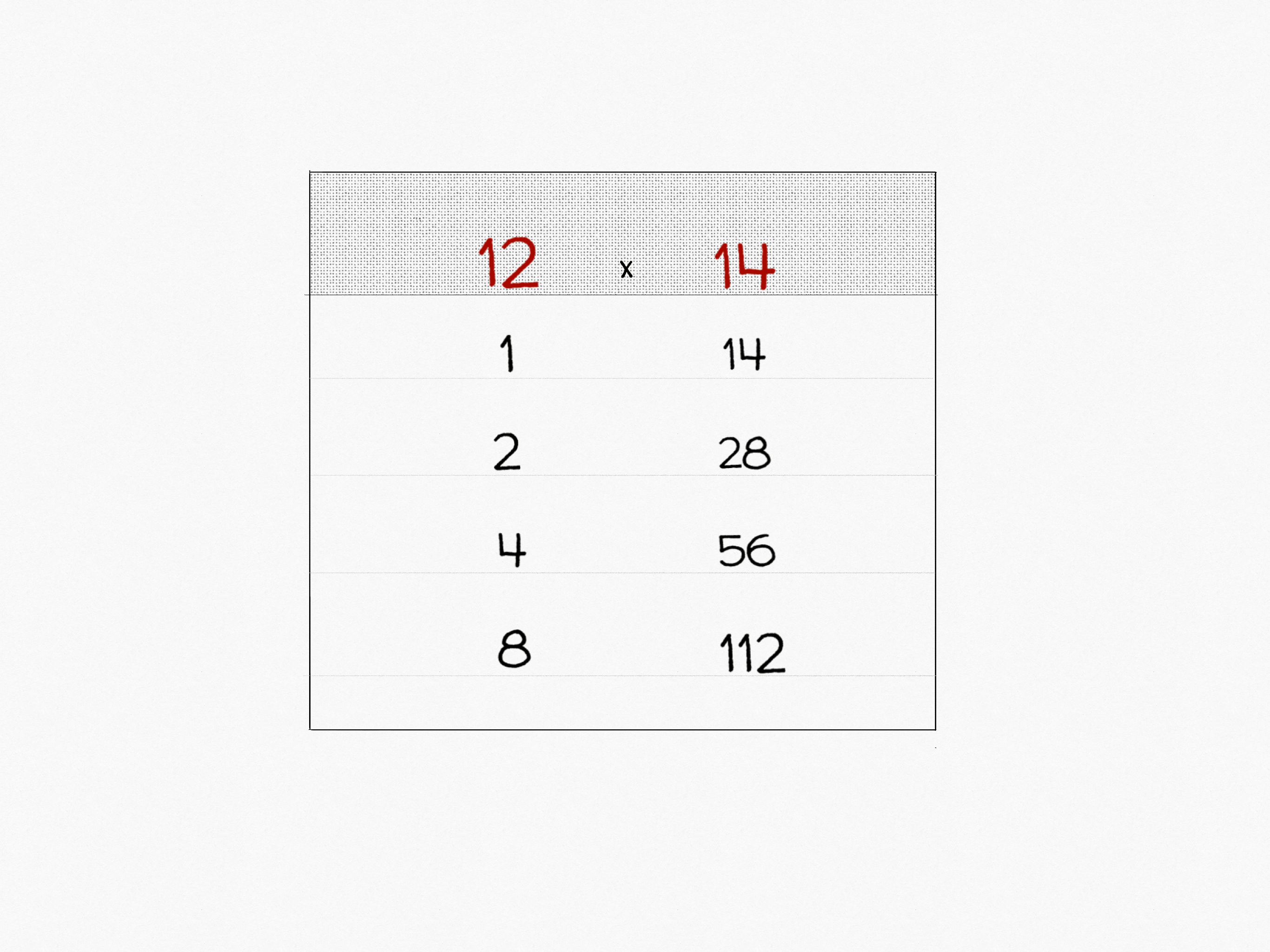On the top, we put the two numbers we want to multiply, below the powers of two (left column) and the number doubled (right column)

So, if we want to multiply 12 and 14, we should draw the two columns and put the multiplicand and the multiplier on the top. Then we write below twelve (we assume it as a multiplier) the powers of two and below fourteen (multiplicand) the numbers 14, 28, 56, 112. There’s no need to go further.

Then we have to select the powers of two that compose the multiplier (so, to have 12, we might add 4 + 8) and we add the corresponding numbers of the second column (in this case we add 56 + 112). The result of the second column is the answer: 168!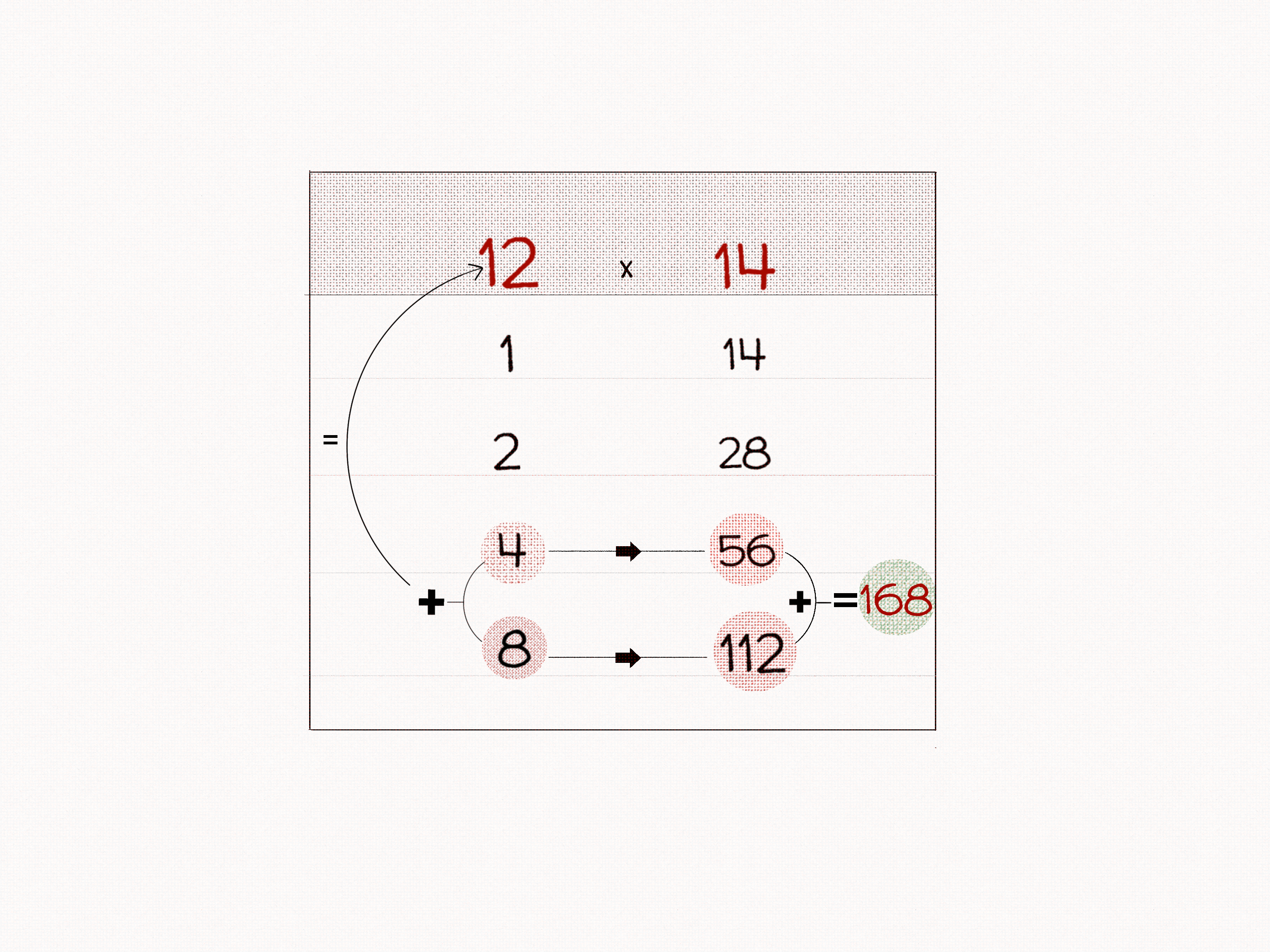Adding the numbers to reach the multiplicand in the left, we add the corresponding on the left and add them

The division is similar, so we will draw again the two columns. Then we write the dividend and the divisor in the place we wrote the multiplier and the multiplicand. Let assume we have to divide 78 by 6. We write our dividend (78) in the first column and the divisor (6) in the second. At this point we shall continue exactly as we did before, writing the powers of two below seventy-eight and doubling six. We stop when the multiples of the divisor reach the dividend.On the top of the graph, we have the dividend and the divisor, below we see the powers of two (left) and the doubling chain of the divisor (right)

Now we just have to add the numbers below 6 until we have the dividend. If we add the corresponding numbers of the other column, we find the answer (8 + 4 + 1 = 13)! Now we can tell - in the ancient Egyptian way that 78 : 6 = 13!Adding the numbers below the divisor to reach the dividend, we add the correspondent ones on the left and add them

What if the number can’t be exactly divided and we have a remainder? It’s easy! Let’s divide 17 with 7: we use our method and we subtract from the lowest number closest to the dividend. So 17 - 14 = 3, and that’s the remainder! Sadly, ancient Egyptians didn’t know the decimals, so the division can’t be accurate like today’s operations, but they still developed their own method to continue solving! They understood that remainders are themselves smaller fractions of the result. So what we have to do is take the remainder and put the divisor as a fraction denominator. If we add it to the result, we know that 17 : 7 = 2 + 3/7! If you don’t believe it, double-check with a calculator!

Fractions were written as a mouth over the denominator. Since the mouth had a value of 1, to have 3/7, we need to find fractions with a numerator of 1 and add them. A possibility is to add 1/14 + 1/42 + 1/3 and we have 3/7, but we could actually be satisfied adding 1/7 + 1/7 + 1/7. These fractions are called unit fractions and we have used them since 1202 AD. It seems that Egyptians have been using them since 2700 BC!## Ancient Egyptians or Computers?

Now, I would like to show you a little trick we’ve just done that you might not have noticed. Let’s focus on the column with powers of two. We can add them to have all the natural numbers. In fact we have 1, 2, 2+1, 4, 4+1, 4+2 and so on. Now, if we take our division and we put a 0 close to the 2, which was excluded, and a I next to the ones we’ve chosen (1, 4, 8) we have this sequence: I0II. We call it binary code, and it’s the same code computers use to do their calculations! We can actually calculate all the divisions with the ancient Egyptian rules and use the binary code to reach the answer.

### 210 : 6 = (1 x 1) + (1 x 2) + (0 x 4) + (0 x 8) + (0 x 16) + (1 x 32) = 35 (in binary 110001)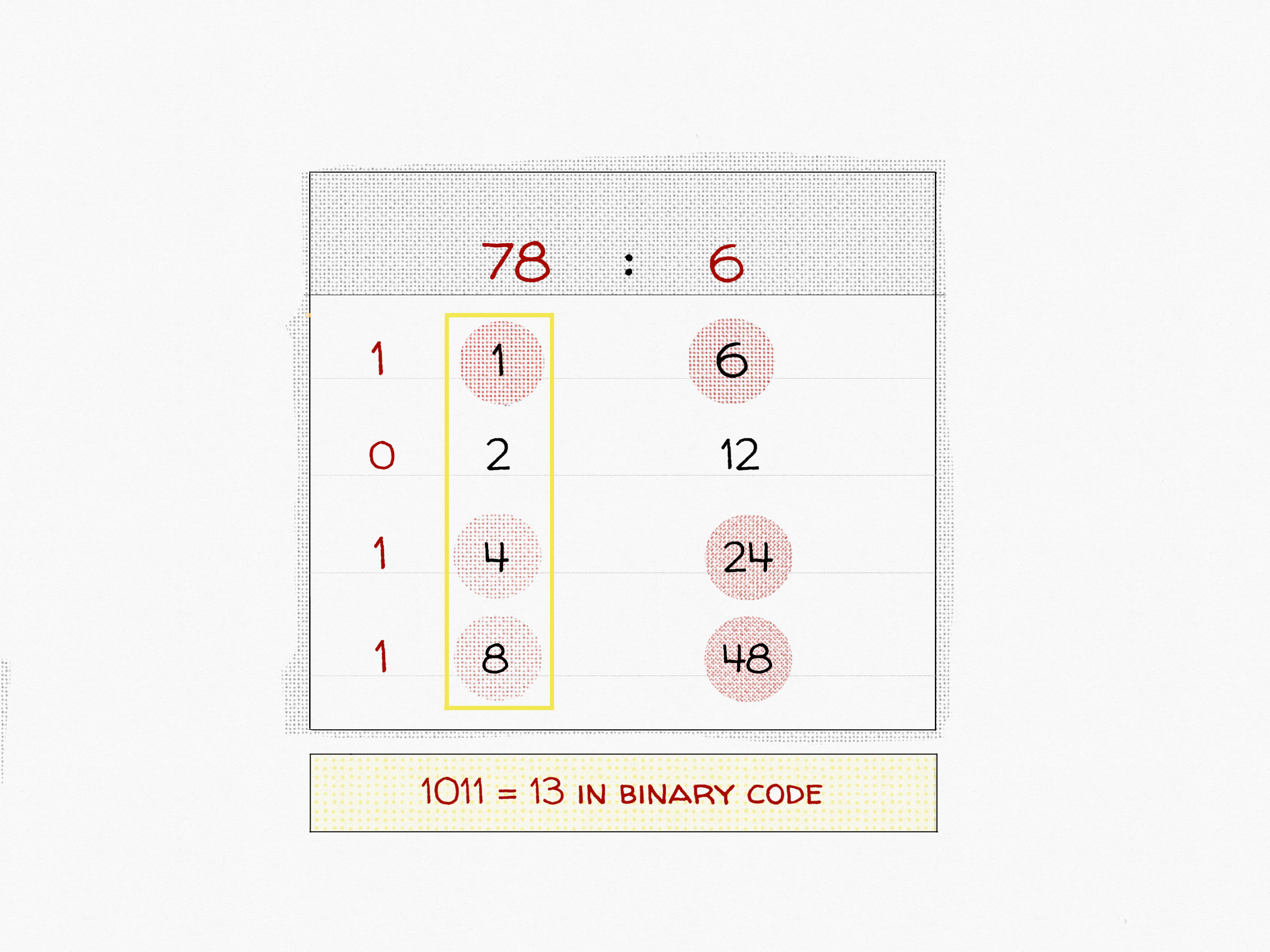The solution of the division, such as the multiplier, can be represented in binary

## The Great Pyramid of Giza: Human Wonder or Alien Knowledge?

The Great Pyramid of Khufu - as it was named after Pharaoh Khufu, which most likely was responsible for its commission - it is the only one of the Seven Wonders of the Ancient World that still remains intact, even if it is the oldest! It was built around 2560 BC and remained the tallest monument ever made for more than 3000 years! We know that Egyptian Pyramids are usually tombs, made to protect powerful people before they embarked upon their journey in the after-death world. There was only one small issue when they opened the pyramid, the tomb was missing its body! Since the Pyramid of Giza was opened in the nineteenth century, there was no dwelling mummy to be found! Usually, great monuments like these are full of inscriptions and information about their host that glorified him, but in this case, the interior passages were completely blank!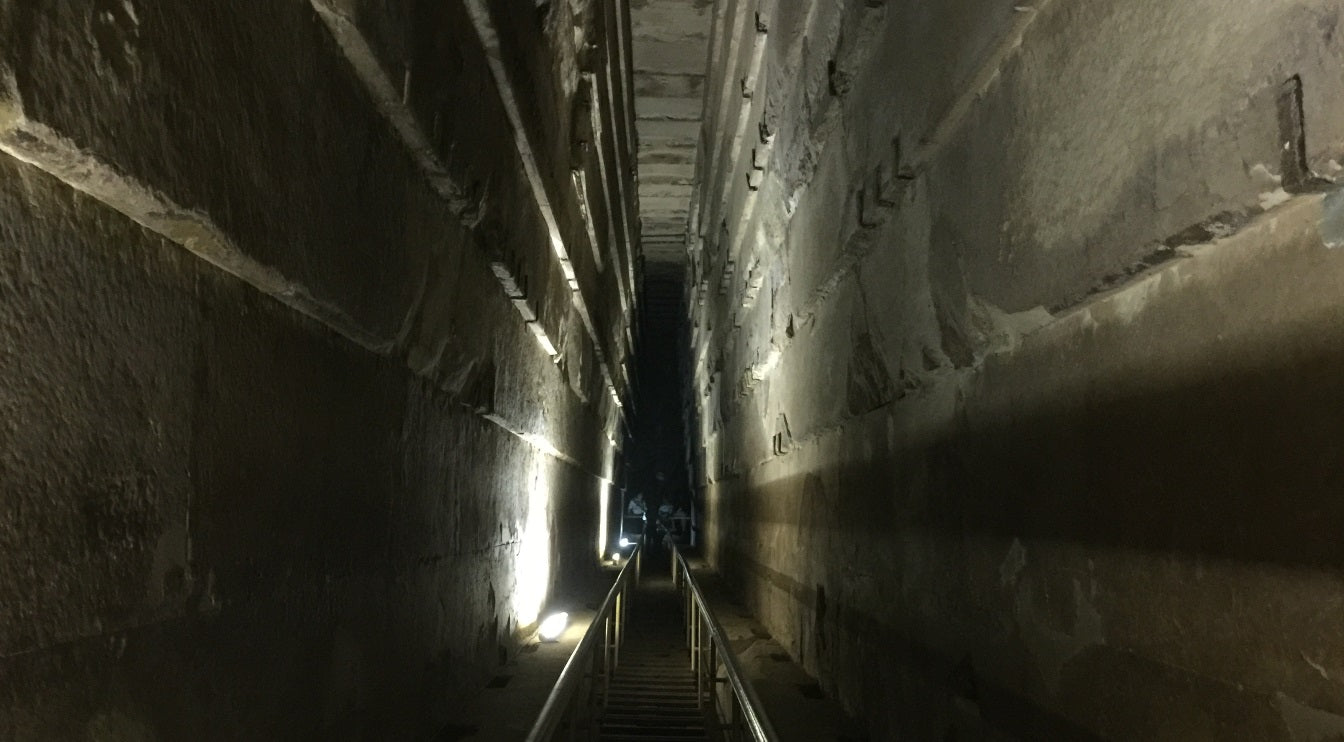The interiors of the Pyramid of Giza

But the most astonishing facts we should consider related to the pyramids are actually the mathematical ones: all the measures of the Great Pyramid are near to perfection, even if made with gigantic irregular blocks in an era with no engineering knowledge! The quadratic base of the pyramid has an average error of 58mm (there are ants that are larger!) and the ratio of the perimeter to height is 2π, even if they didn’t know how to calculate it (or we never found evidence to the contrary)! The alignment of the base is four minutes of arc (1/15 of a degree) wrong in relation to the true north of the earth, which we declared thousands of years later! Some people even support the idea that the latitude where they are located is the same value as the speed of light (and this is actually true, I double checked)!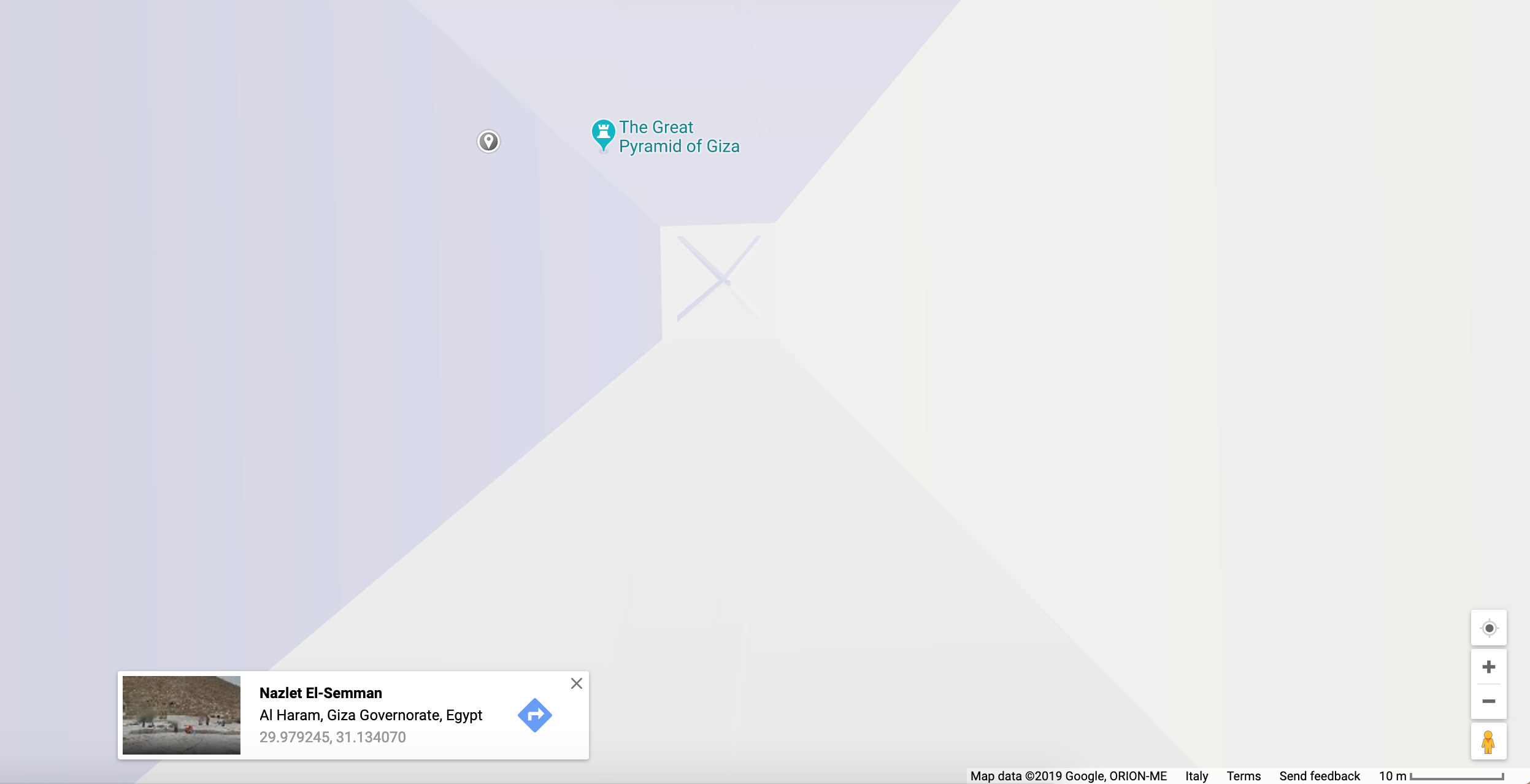The coordinates of the Khufu Pyramid have the same value of the speed of light!

A lot of theories had been made from this information, starting from the alien conspiracy theories to the pure chance. I think that we just don’t know how far the mathematicians of 4000 years ago could have gone, maybe they already knew that earth was round and how to calculate the area of a circle. Who knows what other knowledge have we lost in time?Here you can have a look on our new Egyptian game on Kickstarter!

Liquid error (relatedblogs line 75): internal

### YOU MAY ALSO BE INTERESTED IN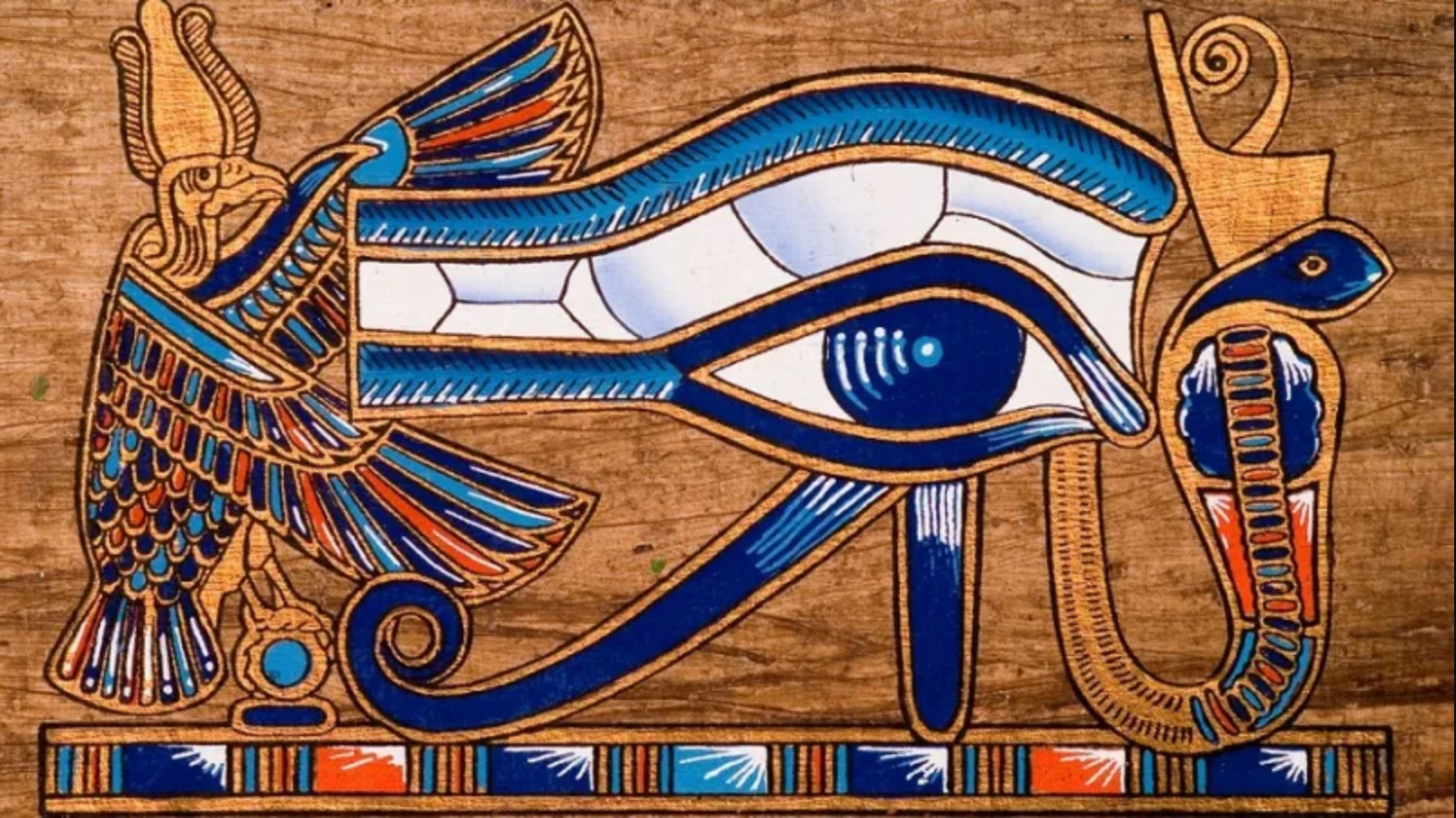##### Ancient Egyptian Math - Part One: Numerals & Hieroglyphics
View of the desert camels and the Pyramids. An overview on Ancient Egypt and Historical evidence of Mathematics in ...##### Now LIVE on Kickstarter: the Thrilling Egyptian Card Game of Deception!
Check out our newest campaign on Kickstarter! KAM'ON is an exciting new card game situated in ancient Egypt where e...##### How to reduce Formaldehyde and VOCs Levels in your Home
VOCs like Formaldehyde can cause Cancer, how can you get rid of them? Photo by Taylor Young You may have heard a...

### RELATED PRODUCTS

• svnfold

This is an inspiring story about mathematic and history! I think very similar and related topic can be found in new book of [https://www.amazon.com/dp/B07XP4C1N6]. The book heavily focused in discussing hidden numerical structure which creates a thematic and semantic network. This network of words is said to be linking every single word in Quranic messages as a ‘mysterious’ pieces of previously unknown information.

• Blane Baclig

Are there any evidence of aliens building the pyramids?